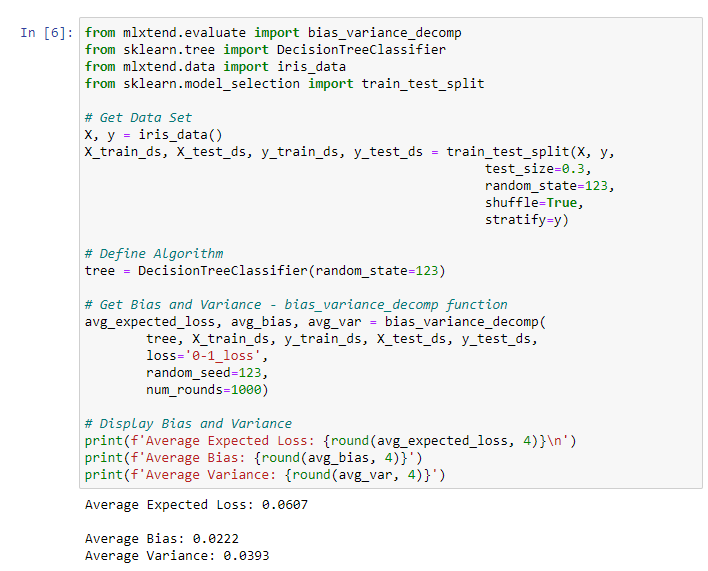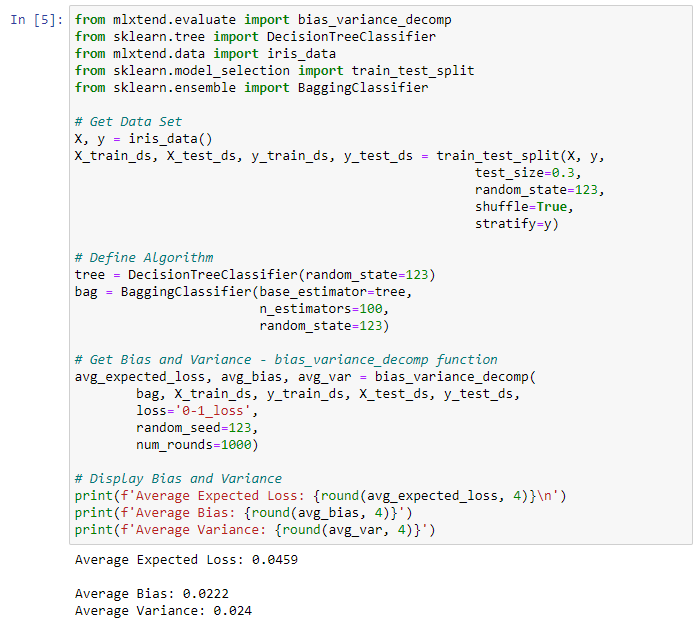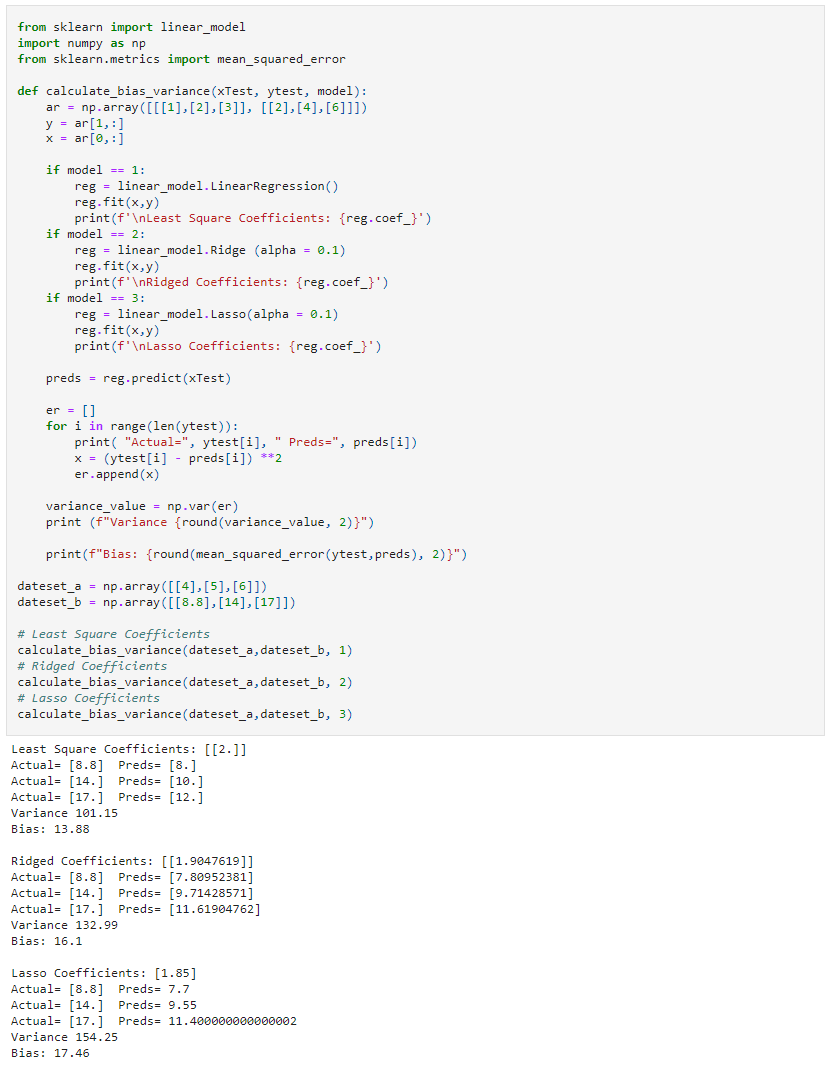﻿ Bias & Variance in Machine Learning: Concepts & Tutorials – BMC Software | Blogs
Machine Learning & Big Data Blog

# Bias & Variance in Machine Learning: Concepts & TutorialsAs machine learning is increasingly used in applications, machine learning algorithms have gained more scrutiny.

With larger data sets, various implementations, algorithms, and learning requirements, it has become even more complex to create and evaluate ML models since all those factors directly impact the overall accuracy and learning outcome of the model. This is further skewed by false assumptions, noise, and outliers.

Machine learning models cannot be a black box. The user needs to be fully aware of their data and algorithms to trust the outputs and outcomes. Any issues in the algorithm or polluted data set can negatively impact the ML model.

This article will examine bias and variance in machine learning, including how they can impact the trustworthiness of a machine learning model.

(New to ML? Read our ML vs AI explainer.)

## What is bias in machine learning?

Bias is a phenomenon that skews the result of an algorithm in favor or against an idea.

Bias is considered a systematic error that occurs in the machine learning model itself due to incorrect assumptions in the ML process.

Technically, we can define bias as the error between average model prediction and the ground truth. Moreover, it describes how well the model matches the training data set:

• A model with a higher bias would not match the data set closely.
• A low bias model will closely match the training data set.

Characteristics of a high bias model include:

• Failure to capture proper data trends
• Potential towards underfitting
• More generalized/overly simplified
• High error rate

## What is variance in machine learning?

Variance refers to the changes in the model when using different portions of the training data set.

Simply stated, variance is the variability in the model prediction—how much the ML function can adjust depending on the given data set. Variance comes from highly complex models with a large number of features.

• Models with high bias will have low variance.
• Models with high variance will have a low bias.

All these contribute to the flexibility of the model. For instance, a model that does not match a data set with a high bias will create an inflexible model with a low variance that results in a suboptimal machine learning model.

Characteristics of a high variance model include:

• Noise in the data set
• Potential towards overfitting
• Complex models
• Trying to put all data points as close as possible

## Underfitting & overfitting

The terms underfitting and overfitting refer to how the model fails to match the data. The fitting of a model directly correlates to whether it will return accurate predictions from a given data set.

• Underfitting occurs when the model is unable to match the input data to the target data. This happens when the model is not complex enough to match all the available data and performs poorly with the training dataset.
• Overfitting relates to instances where the model tries to match non-existent data. This occurs when dealing with highly complex models where the model will match almost all the given data points and perform well in training datasets. However, the model would not be able to generalize the data point in the test data set to predict the outcome accurately.

## Bias vs variance: A trade-off

Bias and variance are inversely connected. It is impossible to have an ML model with a low bias and a low variance.

When a data engineer modifies the ML algorithm to better fit a given data set, it will lead to low bias—but it will increase variance. This way, the model will fit with the data set while increasing the chances of inaccurate predictions.

The same applies when creating a low variance model with a higher bias. While it will reduce the risk of inaccurate predictions, the model will not properly match the data set.

It’s a delicate balance between these bias and variance. Importantly, however, having a higher variance does not indicate a bad ML algorithm. Machine learning algorithms should be able to handle some variance.

We can tackle the trade-off in multiple ways…

Increasing the complexity of the model to count for bias and variance, thus decreasing the overall bias while increasing the variance to an acceptable level. This aligns the model with the training dataset without incurring significant variance errors.

Increasing the training data set can also help to balance this trade-off, to some extent. This is the preferred method when dealing with overfitting models. Furthermore, this allows users to increase the complexity without variance errors that pollute the model as with a large data set.

A large data set offers more data points for the algorithm to generalize data easily. However, the major issue with increasing the trading data set is that underfitting or low bias models are not that sensitive to the training data set. Therefore, increasing data is the preferred solution when it comes to dealing with high variance and high bias models.

This table lists common algorithms and their expected behavior regarding bias and variance:

 Algorithm Bias Variance Linear Regression High Bias Less Variance Decision Tree Low Bias High Variance Bagging Low Bias High Variance (Less than Decision Tree) Random Forest Low Bias High Variance (Less than Decision Tree and Bagging)

## Bias & variance calculation example

Let’s put these concepts into practice—we’ll calculate bias and variance using Python.

The simplest way to do this would be to use a library called mlxtend (machine learning extension), which is targeted for data science tasks. This library offers a function called bias_variance_decomp that we can use to calculate bias and variance.

We will be using the Iris data dataset included in mlxtend as the base data set and carry out the bias_variance_decomp using two algorithms: Decision Tree and Bagging.

### Decision tree example

```from mlxtend.evaluate import bias_variance_decomp
from sklearn.tree import DecisionTreeClassifier
from mlxtend.data import iris_data
from sklearn.model_selection import train_test_split

# Get Data Set
X, y = iris_data()
X_train_ds, X_test_ds, y_train_ds, y_test_ds = train_test_split(X, y,
test_size=0.3,
random_state=123,
shuffle=True,
stratify=y)

# Define Algorithm
tree = DecisionTreeClassifier(random_state=123)

# Get Bias and Variance - bias_variance_decomp function
avg_expected_loss, avg_bias, avg_var = bias_variance_decomp(
tree, X_train_ds, y_train_ds, X_test_ds, y_test_ds,
loss='0-1_loss',
random_seed=123,
num_rounds=1000)

# Display Bias and Variance
print(f'Average Expected Loss: {round(avg_expected_loss, 4)}n')
print(f'Average Bias: {round(avg_bias, 4)}')
print(f'Average Variance: {round(avg_var, 4)}')
```

Result:### Bagging example

```from mlxtend.evaluate import bias_variance_decomp
from sklearn.tree import DecisionTreeClassifier
from mlxtend.data import iris_data
from sklearn.model_selection import train_test_split
from sklearn.ensemble import BaggingClassifier

# Get Data Set
X, y = iris_data()
X_train_ds, X_test_ds, y_train_ds, y_test_ds = train_test_split(X, y,
test_size=0.3,
random_state=123,
shuffle=True,
stratify=y)

# Define Algorithm
tree = DecisionTreeClassifier(random_state=123)
bag = BaggingClassifier(base_estimator=tree,
n_estimators=100,
random_state=123)

# Get Bias and Variance - bias_variance_decomp function
avg_expected_loss, avg_bias, avg_var = bias_variance_decomp(
bag, X_train_ds, y_train_ds, X_test_ds, y_test_ds,
loss='0-1_loss',
random_seed=123,
num_rounds=1000)

# Display Bias and Variance
print(f'Average Expected Loss: {round(avg_expected_loss, 4)}n')
print(f'Average Bias: {round(avg_bias, 4)}')
print(f'Average Variance: {round(avg_var, 4)}')
```

Result:Each of the above functions will run 1,000 rounds (num_rounds=1000) before calculating the average bias and variance values. There, we can reduce the variance without affecting bias using a bagging classifier. The higher the algorithm complexity, the lesser variance.

In the following example, we will have a look at three different linear regression models—least-squares, ridge, and lasso—using sklearn library. Since they are all linear regression algorithms, their main difference would be the coefficient value.

We can see those different algorithms lead to different outcomes in the ML process (bias and variance).

```from sklearn import linear_model
import numpy as np
from sklearn.metrics import mean_squared_error

def calculate_bias_variance(xTest, ytest, model):
ar = np.array([[,,], [,,]])
y = ar[1,:]
x = ar[0,:]

if model == 1:
reg = linear_model.LinearRegression()
reg.fit(x,y)
print(f'nLeast Square Coefficients: {reg.coef_}')
if model == 2:
reg = linear_model.Ridge (alpha = 0.1)
reg.fit(x,y)
print(f'nRidged Coefficients: {reg.coef_}')
if model == 3:
reg = linear_model.Lasso(alpha = 0.1)
reg.fit(x,y)
print(f'nLasso Coefficients: {reg.coef_}')

preds = reg.predict(xTest)

er = []
for i in range(len(ytest)):
print( "Actual=", ytest[i], " Preds=", preds[i])
x = (ytest[i] - preds[i]) **2
er.append(x)

variance_value = np.var(er)
print (f"Variance {round(variance_value, 2)}")

print(f"Bias: {round(mean_squared_error(ytest,preds), 2)}")

dateset_a = np.array([,,])
dateset_b = np.array([[8.8],,])

# Least Square Coefficients
calculate_bias_variance(dateset_a,dateset_b, 1)
# Ridged Coefficients
calculate_bias_variance(dateset_a,dateset_b, 2)
# Lasso Coefficients
calculate_bias_variance(dateset_a,dateset_b, 3)
```

Result:## Considering bias & variance is crucial

Bias and variance are two key components that you must consider when developing any good, accurate machine learning model.

• Bias creates consistent errors in the ML model, which represents a simpler ML model that is not suitable for a specific requirement.
• On the other hand, variance creates variance errors that lead to incorrect predictions seeing trends or data points that do not exist.

Users need to consider both these factors when creating an ML model. Generally, your goal is to keep bias as low as possible while introducing acceptable levels of variances. This can be done either by increasing the complexity or increasing the training data set.

In this balanced way, you can create an acceptable machine learning model.

This e-book teaches machine learning in the simplest way possible. This book is for managers, programmers, directors – and anyone else who wants to learn machine learning. We start with very basic stats and algebra and build upon that.

These postings are my own and do not necessarily represent BMC's position, strategies, or opinion.

See an error or have a suggestion? Please let us know by emailing blogs@bmc.com.

### BMC Bring the A-Game

From core to cloud to edge, BMC delivers the software and services that enable nearly 10,000 global customers, including 84% of the Forbes Global 100, to thrive in their ongoing evolution to an Autonomous Digital Enterprise.